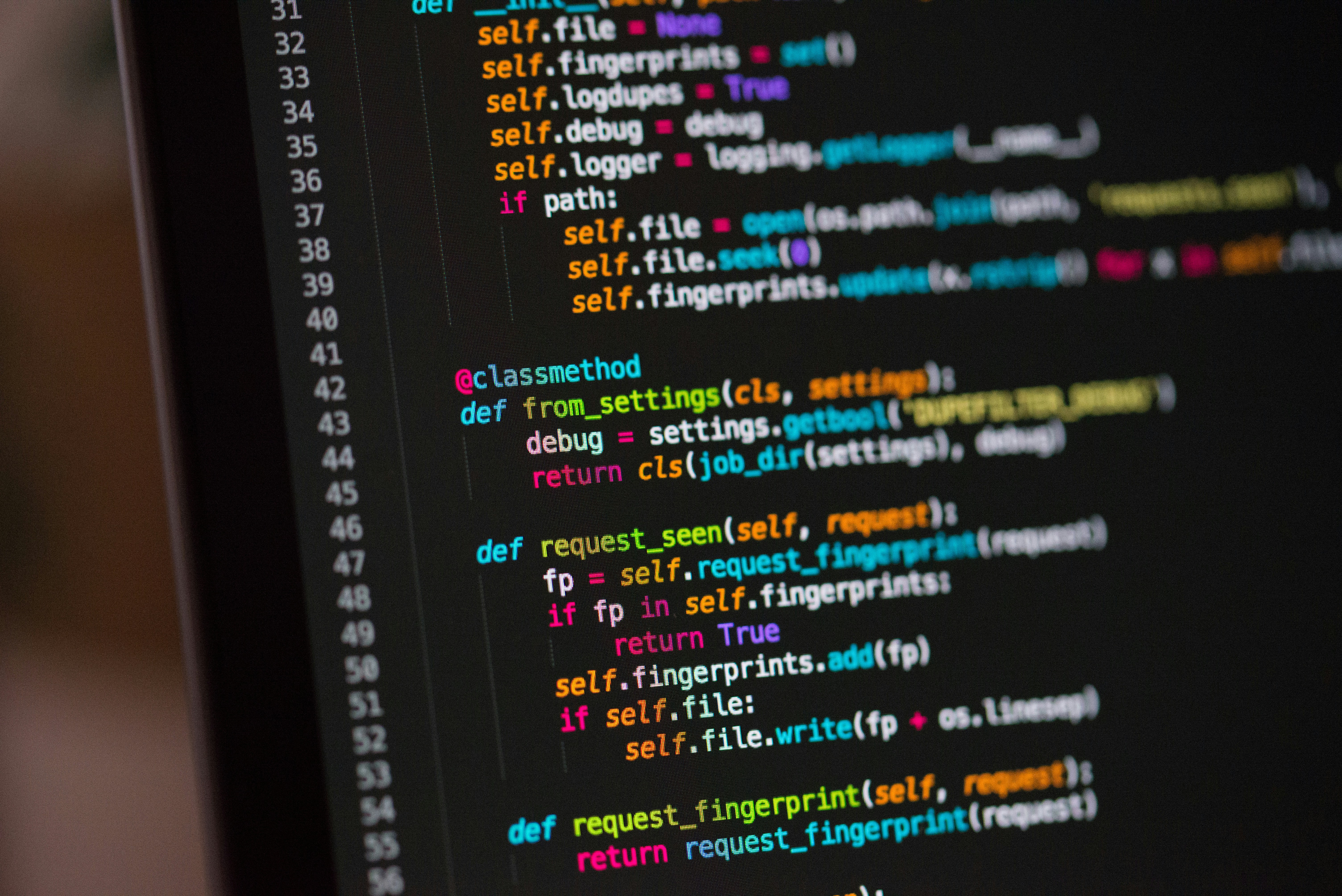Hackerrank Java Interface Solution

# Hackerrank Java Interface SolutionA Java interface can only contain method signatures and fields. The interface can be used to achieve polymorphism. In this problem, you will practice your knowledge on interfaces.

You are given an interface AdvancedArithmetic which contains a method signature int divisor_sum(int n). You need to write a class called MyCalculator which implements the interface.

divisorSum function just takes an integer as input and return the sum of all its divisors. For example divisors of 6 are 1, 2, 3 and 6, so divisor_sum should return 12. The value of n will be at most 1000.

Read the partially completed code in the editor and complete it. You just need to write the MyCalculator class only. Your class shouldn't be public.

Sample Input

6


Sample Output

I implemented: AdvancedArithmetic
12


Explanation

Divisors of 6 are 1,2,3 and 6. 1+2+3+6=12.

### Solution in java8

Approach 1.

class MyCalculator implements AdvancedArithmetic {
public int divisor_sum(int n) {
int sum=0;
for(int i=1;i<=n;i++) {
if(n%i==0)
sum+=i;
}
return sum;
}
}

Approach 2.

class MyCalculator implements AdvancedArithmetic{

public int divisor_sum(int n){
int sum = 1;
for (int i=2;i<=n;i++){
if (n%i == 0){
sum = sum + i;
}
}
return sum;
}
}

Approach 3.

//Write your code here
class MyCalculator implements AdvancedArithmetic{
public int divisor_sum(int n){
int sum = 0;
for(int i = 1; i <= n; i++){
if(n % i == 0){
sum+= i;
}
}

return sum;
}
}